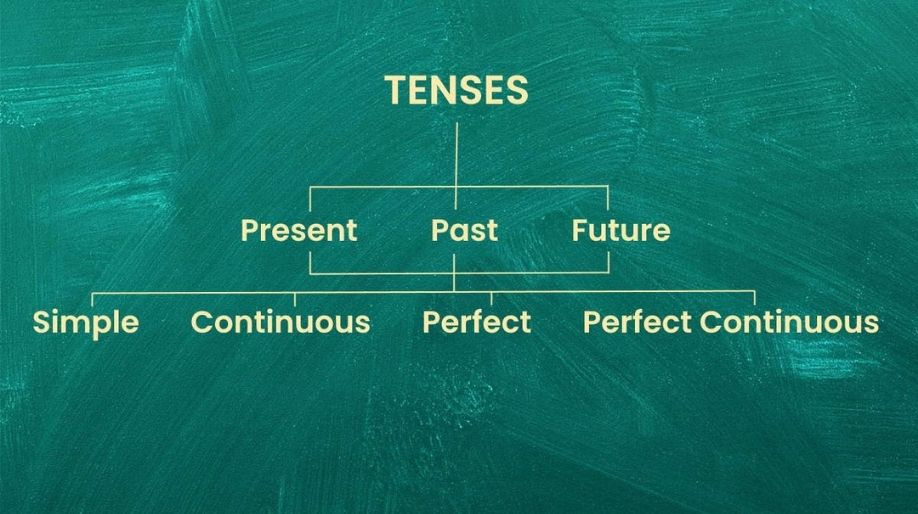Register for Maths, Science, English, Reasoning Olympiad Exams Register here | Book Free Demo for Live Olympiad Classes here | Check Olympiad Exam Dates here | Buy Practice Papers for IEO, IOEL, HEO, IOEL etc here | Login here to participate in all India free mock test on every Saturday

Simple TensesBased on the time of action, tenses are of three types.

• Past tense
• Present tense
• Future tense

Simple past tense:
Past tense refers to the actions that have already happened.

Examples,
• I went for long drive yesterday.
• He watched television all night.
• He worked in a bank.

Simple present tense:
Present tense refers to the actions that take place regularly.

Examples,
• I sleep early.
• We go for swimming on Sundays.
• The Earth revolves around the Sun.

Simple future tense:
Future tense refers to the actions that are expected to happen in future.

Examples,
• I will eat a mango.
• I will go for a walk.
• He will teach you English.

Exercise

Choose the correct tense.

1. I went to market.
a. Simple past tense
b. Simple present tense
c. Simple future tense
d. None of the above

2. I will call you tomorrow.
a. Simple present tense
b. Simple past tense
c. Simple future tense
d. None of the above

3. I play football very well.
a. Simple past tense
b. Simple present tense
c. Simple future tense
d. None of the above

4. Mr Ravi taught us English.
a. Simple present tense
b. Simple past tense
c. Simple future tense
d. None of the above

5. My father goes to temple every day.
a. Simple present tense
b. Simple past tense
c. Simple future tense
d. None of the above

6. I will spend holidays in Shimla.
a. Simple past tense
b. Simple present tense
c. Simple future tense
d. None of the above

7. He called me last night.
a. Simple future tense
b. Simple present tense
c. Simple past tense
d. None of the above

8. The policeman caught the thief.
a. Simple past tense
b. Simple present tense
c. Simple future tense
d. None of the above

9. The climate in my city is very hot.
a. Simple past tense
b. Simple present tense
c. Simple future tense
d. None of the above

10. The jury will announce its verdict today.
a. Simple future tense
b. Simple past tense
c. Simple present tense
d. None of the above